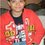Is it a fallacy ??

Recently, I was doodling in my rough notebook and found something strange:

Let x belong to N (natural no.)

$x^{2}$ = $x^{2}$

$\Rightarrow$ $x^{2}$ = $x+x+x+x+x+x....(x times)$

(Differentiating on both sides)

$2x$ = $1+1+1+1+1+1+1....(x times)$

$\Rightarrow 2x = x$

Where was I wrong ??Note by Ashwin Upadhyay
4 years, 4 months ago

This discussion board is a place to discuss our Daily Challenges and the math and science related to those challenges. Explanations are more than just a solution — they should explain the steps and thinking strategies that you used to obtain the solution. Comments should further the discussion of math and science.

When posting on Brilliant:

• Use the emojis to react to an explanation, whether you're congratulating a job well done , or just really confused .
• Ask specific questions about the challenge or the steps in somebody's explanation. Well-posed questions can add a lot to the discussion, but posting "I don't understand!" doesn't help anyone.
• Try to contribute something new to the discussion, whether it is an extension, generalization or other idea related to the challenge.
• Stay on topic — we're all here to learn more about math and science, not to hear about your favorite get-rich-quick scheme or current world events.

MarkdownAppears as
*italics* or _italics_ italics
**bold** or __bold__ bold

- bulleted
- list

• bulleted
• list

1. numbered
2. list

1. numbered
2. list
Note: you must add a full line of space before and after lists for them to show up correctly
paragraph 1

paragraph 2

paragraph 1

paragraph 2

> This is a quote
This is a quote
# I indented these lines
# 4 spaces, and now they show
# up as a code block.

print "hello world"
# I indented these lines
# 4 spaces, and now they show
# up as a code block.

print "hello world"
MathAppears as
Remember to wrap math in $$...$$ or $...$ to ensure proper formatting.
2 \times 3 $2 \times 3$
2^{34} $2^{34}$
a_{i-1} $a_{i-1}$
\frac{2}{3} $\frac{2}{3}$
\sqrt{2} $\sqrt{2}$
\sum_{i=1}^3 $\sum_{i=1}^3$
\sin \theta $\sin \theta$
\boxed{123} $\boxed{123}$

Sort by:

When you are defining x^2=x+x+..+x(x times), you are tacitly defining the domain of f(x) to be N, the set of natural numbers. However, f(x) cannot be differentiable in the domain of N. This is because, if a function f defined from a domain D (a subset of R, the set of reals) to R (the set of reals) has to be differentiable at a real point c, then a necessary criterion is that c has to be a limit point of D and c has to be an element of D itself. In other words, c has to be a member of D such that every arbitrarily small neighbourhood of c has an element of D other than c. But no natural number is a cluster point of the set N. Here lies the fallacy.

- 4 years, 3 months ago

When you are differentiating on both sides, you assumed that in x times the x is constant. Also you can't talk abt differention, of non continuous function

- 4 years, 4 months ago

I couldn't understand your first reason.

- 4 years, 4 months ago

Actually you cannot differentiate the function f(x) = $x^{2}$ when you have selected the Domain as Natural numbers . After all , the Natural numbers as a domain will comprise just discrete points , so it'll not be differentiable.

- 4 years, 4 months ago

Very good. He is fooling.

- 4 years, 4 months ago

Firstly I differentiated it generally but in that case i couldn't write the no. x times as x could be fraction or 0. Thank you now i have understood.

- 4 years, 4 months ago

Anytime . Btw there was a question on this fallacy posted by @Sandeep Bhardwaj sir , I'm not able to find it . I'll give you the link if I'm able to find it .

- 4 years, 4 months ago

In fact i found a whole set of such apparent fallacies

- 4 years, 4 months ago

Wow . You've been busy :P

- 4 years, 4 months ago

BTW i just found another note on this

- 4 years, 4 months ago